SYLLABUS  Previous: 6.2.1 The Black-Scholes equation  Up: 6.2 Methods for American  Next: 6.3 Computer quiz

6.2.2 Solution of the variational inequality using finite elements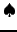[ SLIDE complementary problem - scheme 2 || same VIDEO as previous section: modem - LAN - DSL]

To satisfy the Black-Scholes and the free-boundary inequalities simultaneously, we follow the same method that is generally used when dealing with obstacle problems. Both inequalities are first cast into a complementary problem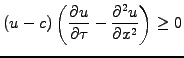(6.2.2#eq.1)

which has to be satisfied subject the free-boundary condition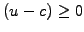. Following the spirit of sect.5.3 and using Galerkin's method to solve an equivalent variational principle, choose a test function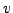that is sufficiently general'' and satisfies the same constraints as the solution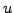. By construction, this satisfies the inequality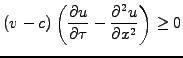(6.2.2#eq.2)

Integrate both inequalities over the domain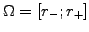where a solution is sought and subtract (6.2.2#eq.1) from (6.2.2#eq.2) to formulate an equivalent variational principle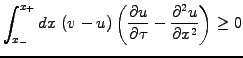(6.2.2#eq.3)

This now depends only implicitly on the condition (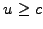) through the choice of the test function. Integrate by parts the second order derivative with a vanishing surface term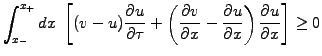(6.2.2#eq.4)

Decompose the solution and test functions in a series of finite element roof-tops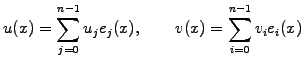(6.2.2#eq.5)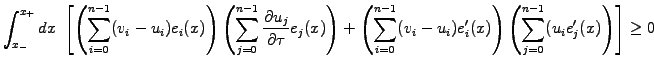(6.2.2#eq.6)

Using the notation for the overlap integrals in (5.3.1#eq.10), this yields the inequality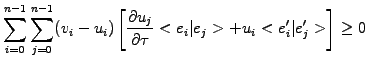(6.2.2#eq.7)

Discretize time with small steps using a forward difference for the first term and a partly implicit evaluation for the second, writing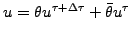and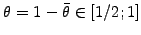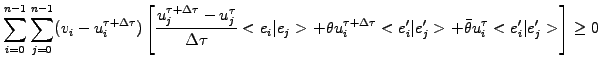(6.2.2#eq.8)

which finally yields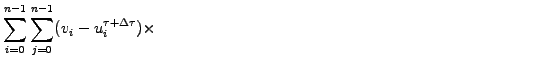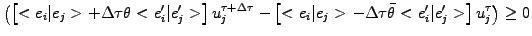and remains subject to the free-boundary condition. As for obstacle problems in general, it is possible to show that both conditions can only be satisfied if the inequality (6.2.2#eq.9) satisfies the equality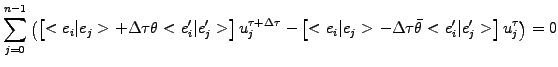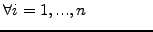(6.2.2#eq.9)

where the solution satisfies the free-boundary condition. The problem is finally solved using an iterative method called projected successive over-relaxation (SSOR), where the solution is sucessively improved from an initial guess-in a manner that guarantees that the free-boundary conditionis always satisfied. Note the strong resemblance with the finite element scheme for European options, which uses exactly the same matrices. The European and American problems are therefore conveniently implemented into the same program, changing only the boundary conditions and the SSOR solver to account for the different exercise styles. Using the same header and footer as in sect.4.4.2 to transform between financial and log-normal variables, the entire scheme has been implemented in FEMSolution.java as
//--- CONSTRUCT MATRICES
BandMatrix a = new BandMatrix(3, f.length);      // Linear problem
BandMatrix b = new BandMatrix(3, f.length);      //  a*fp=b*f=c
double[]   c = new double[f.length];
double htm = dxk*(1-tune)/4;                     // Quadrature coeff
double htp = dxk*(1+tune)/4;
double halpha = dtau/dxk;                        // PDE coefficient
for (int i=0; i<=n; i++) {
a.setL(i,   htm -halpha* theta    );
a.setD(i,2*(htp +halpha* theta   ));
a.setR(i,   htm -halpha* theta    );
b.setL(i,   htm -halpha*(theta-1) );
b.setD(i,2*(htp +halpha*(theta-1)));
b.setR(i,   htm -halpha*(theta-1) );
}
c=b.dot(fm);
a.setL(0,0.);a.setD(0,1.);a.setR(0,0.);c=0;   // First equation idle

//--- BC + SOLVE
if (scheme.equals(vmarket.EUNORM)) {             // European option
a.setL(1, 0.);a.setD(1,1.);a.setR(1,0.);       //   in-money: Dirichlet
c=Math.exp(0.5*k2m1*xk0+0.25*k2m1*k2m1*tau);
a.setL(n,-1.);a.setD(n,1.);a.setR(n,0.);c[n]=0.; // out-money: Neuman
f=a.ssor3(c,fm);                               // conventional-SSOR
y0=strike*Math.exp(-rate*time);

} else if (scheme.equals(vmarket.AMNORM)) {      // American option
double[] min = new double[f.length];           // Obstacle
double[] max = new double[f.length];
for (int i=0; i<=n; i++) {
xk=xk0+(i-1)*dxk;
min[i]=Math.exp((0.25*k2m1*k2m1 +k1)*tau) *
Math.max(0., Math.exp(0.5*k2m1*xk) -
Math.exp(0.5*k2p1*xk)  );
max[i]=Double.POSITIVE_INFINITY;
fm[i]=Math.max(min[i],f[i]);                 //   IC interp error
}
a.setL(1, 0.);a.setD(1,1.);a.setR(1,0.);       // In-money: Dirichlet
c=Math.max(min,Math.exp(0.5*k2m1*xk0+0.25*k2m1*k2m1*tau));
a.setL(n,-1.);a.setD(n,1.);a.setR(n,0.);c[n]=0.; //out-money: Neuman
double precision = strike*Math.pow(10.,-6);      // relativ.to strike
int maxIter = 30;
double w = 1.2;                                // relaxation parameter
f=a.ssor3(c,fm,min,max,precision,w,maxIter);   // projected-SSOR
fp=strike;
}
The code has intentionally been restricted for the case of American put options, leaving the complete implementation with call options to the reader (exercise 6.06). Note that the terminal and boundary conditions have been specified here in log-normal variables (4.4.2#eq.4), using the same definitions as for the finite differences in sect.4.4.2

SYLLABUS  Previous: 6.2.1 The Black-Scholes equation  Up: 6.2 Methods for American  Next: 6.3 Computer quiz